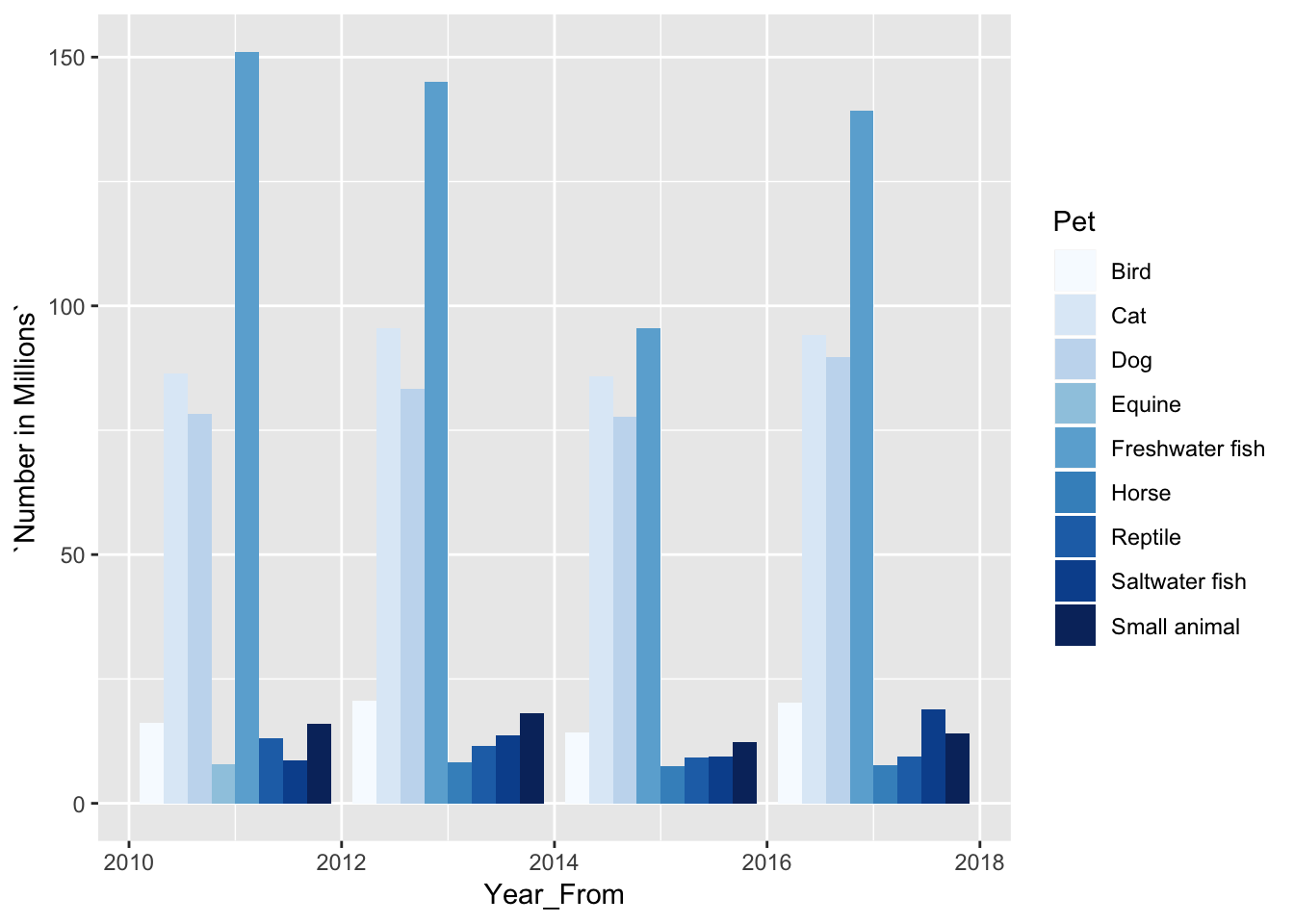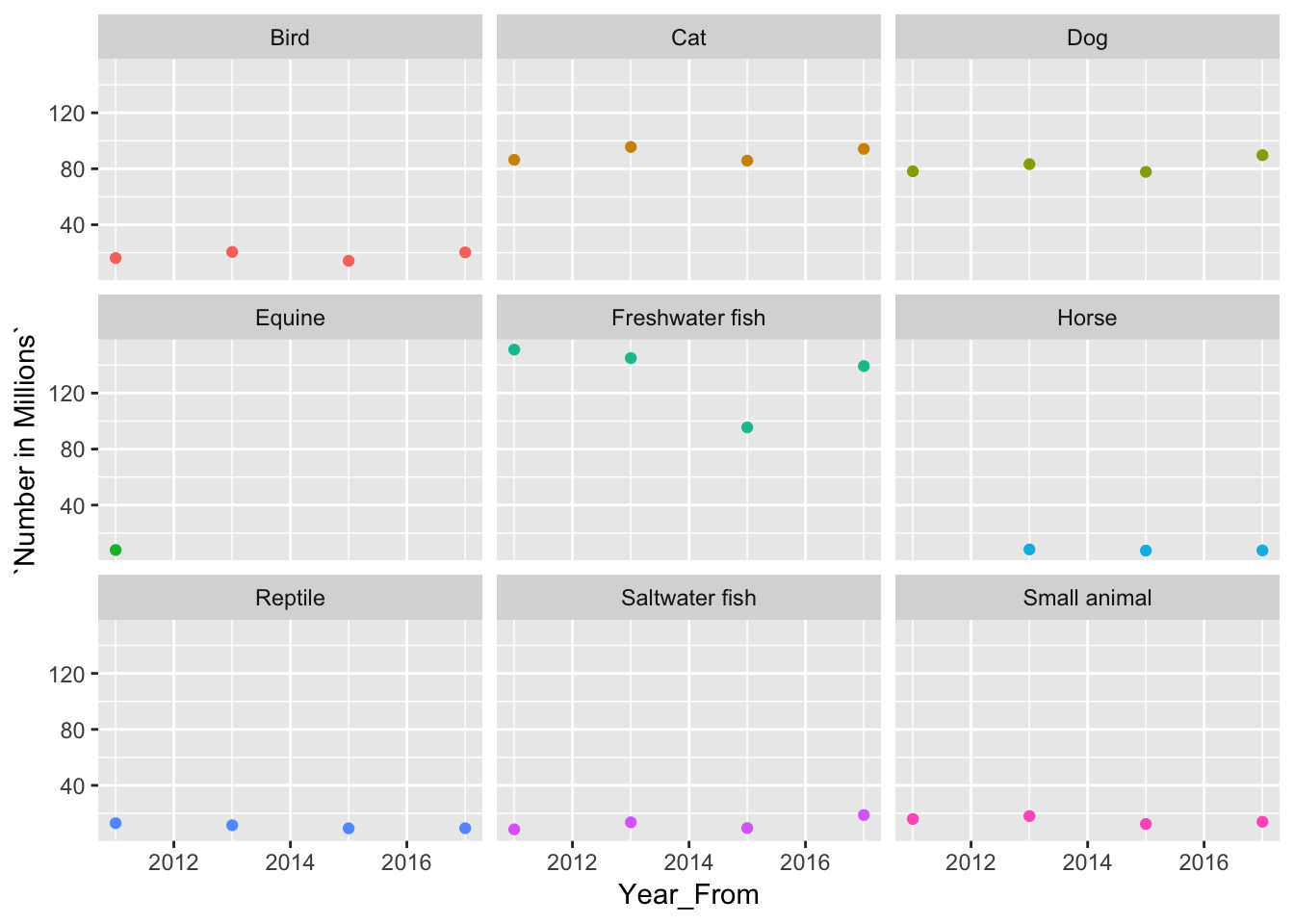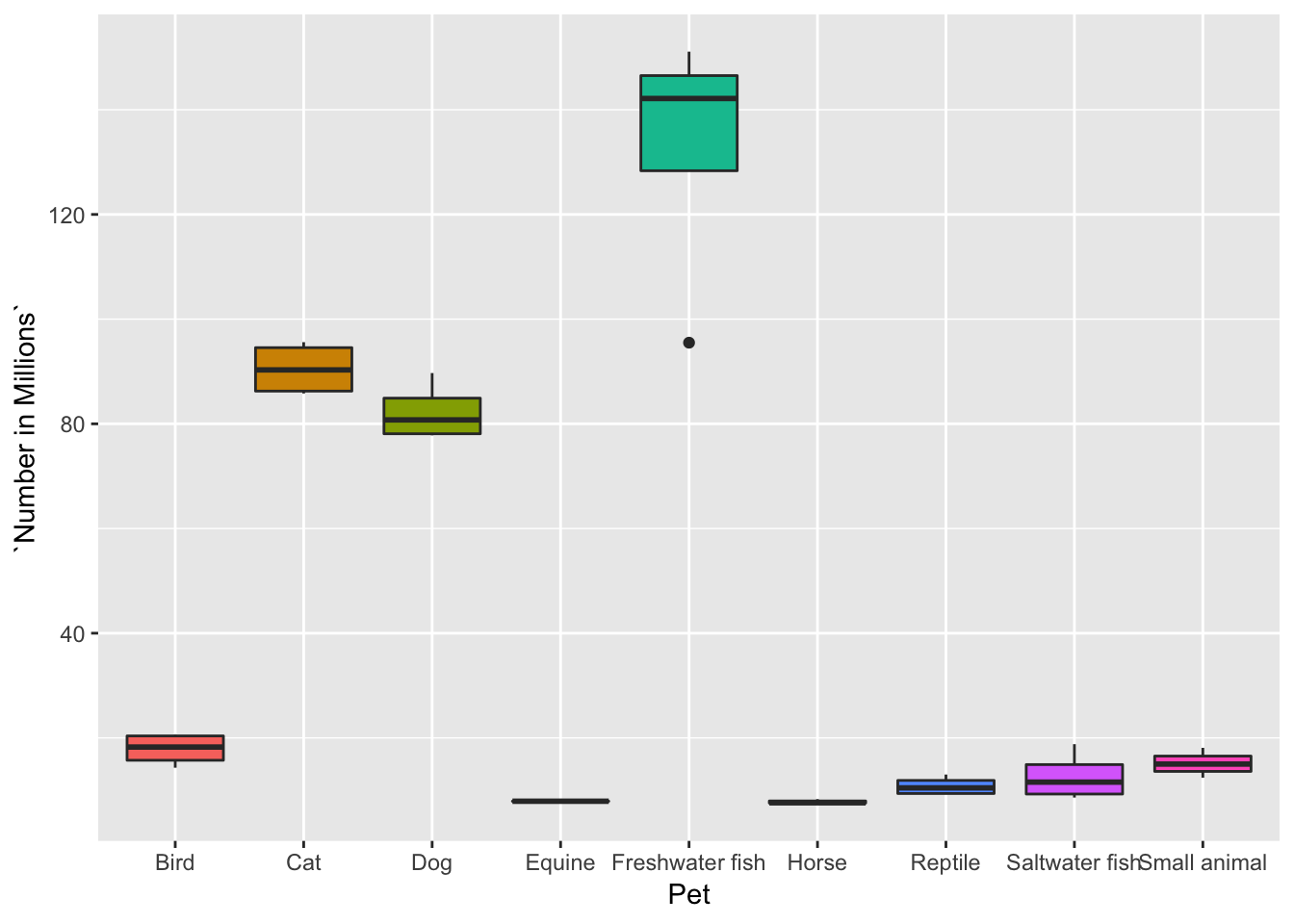# R for "Paws"With the help of 2 R-Ninja Eighth Graders, Tara and Ben who have been R-ing since they were 5th graders, I recently taught Coding in R: Information Visualized class (Feb 23rd - March 2019) to Middle Graders (Grades 6-8) for Saturday Academy, a non-profit organisation. We donated all proceeds from teaching the class to Family Dog Shelter, Portland, OR and Oregon Humane Society, Portland, OR, and we will continue to R for Paws by donating all proceeds from teaching R to animal shelters.

Here’s a brief of what we taught, and I should have the entire class materials posted on github by end of June 2019.

The 3 key objectives of the Coding in R: Information Visualized class:

#### Objective 1: Understand and Construct data sets using vectors and tibbles:

Type_of_Pets <- c("Freshwater_Fish", "Cat", "Dog", "Bird",
"Saltwater fish", "small animal", "Reptile", "Horse")
Number_of_Pets <- c(139.3, 94.2, 89.7, 20.3,
18.8, 14, 9.4, 7.6)
Total_Pets <- tibble(Type_of_Pets, Number_of_Pets)

# View Total_Pets
Total_Pets
## # A tibble: 8 x 2
##   Type_of_Pets    Number_of_Pets
##   <chr>                    <dbl>
## 1 Freshwater_Fish          139.
## 2 Cat                       94.2
## 3 Dog                       89.7
## 4 Bird                      20.3
## 5 Saltwater fish            18.8
## 6 small animal              14
## 7 Reptile                    9.4
## 8 Horse                      7.6
# View the structure of the data. What type of variables do you have?
str(Total_Pets)
## Classes 'tbl_df', 'tbl' and 'data.frame':    8 obs. of  2 variables:
##  $Type_of_Pets : chr "Freshwater_Fish" "Cat" "Dog" "Bird" ... ##$ Number_of_Pets: num  139.3 94.2 89.7 20.3 18.8 ...
##### Based on Understanding Objective 1, move to reading local excel/csv/rds files. Data from:
Pet_US_Stats <- readxl::read_excel("~/Documents/sunitakenner/Product_Data/Day2_Pet_Data.xlsx")

#Different ways you can look at data
glimpse(Pet_US_Stats)
## Observations: 32
## Variables: 5
## $Pet <chr> "Freshwater fish", "Cat", "Dog", "Bird", "… ##$ Number in Millions  <dbl> 139.3, 94.2, 89.7, 20.3, 18.8, 14.0, 9.4, …
## $Households that Own <dbl> 12.5, 47.1, 60.2, 7.9, 2.5, 6.7, 4.7, 2.6,… ##$ Year_From             <dttm> 2017-01-01, 2017-01-01, 2017-01-01, 2017-…
## $Year_To <dttm> 2018-01-01, 2018-01-01, 2018-01-01, 2018-… skimr::skim(Pet_US_Stats) ## Skim summary statistics ## n obs: 32 ## n variables: 5 ## ## ── Variable type:character ────────────────────────────────────────────────────────── ## variable missing complete n min max empty n_unique ## Pet 0 32 32 3 15 0 9 ## ## ── Variable type:numeric ──────────────────────────────────────────────────────────── ## variable missing complete n mean sd p0 p25 p50 p75 ## Households that Own 0 32 32 16.56 19.6 0.7 4.15 6.4 20.45 ## Number in Millions 0 32 32 46.21 46.73 7.5 11 17.15 85.95 ## p100 hist ## 60.2 ▇▂▁▁▁▁▁▁ ## 151.1 ▇▁▁▁▃▁▁▁ ## ## ── Variable type:POSIXct ──────────────────────────────────────────────────────────── ## variable missing complete n min max median n_unique ## Year_From 0 32 32 2011-01-01 2017-01-01 2014-01-01 4 ## Year_To 0 32 32 2012-01-01 2018-01-01 2015-01-01 4 #### Objective 2: Visualize the dataset using ggplot2 ggplot(Pet_US_Stats) + geom_col(mapping = aes(x =Year_From , y = Number in Millions, fill = Pet), position = "dodge") + scale_fill_brewer()##### The facet_wrap() power ggplot(Pet_US_Stats) + geom_point(aes(x = Year_From, y = Number in Millions, color = Pet)) + facet_wrap(~Pet) + theme(legend.position = "none")##### Visualizing median, range: The geom_boxplot() power ggplot(Pet_US_Stats) + geom_boxplot(mapping = aes(x = Pet, y = Number in Millions, fill = Pet)) + theme(legend.position = "none")#### Objective 3: Learn the basics of writing functions in R (mean, median, range) The middle schoolers who attended this class were well acquainted with the Math for deriving (mean, median, range). Mapping the math to the functions in R as demonstrated below proved to be a fun learning experience for the kids. They loved it (Please see feedback at the end of the post for the class)!!!! Two middle schoolers volunteered to write their own mean and range functions. ##### The mean function by Martin (A 7th Grader) #Create a numerical vector of length 5 test_vector <- c(4,5,7,1,6) #Add the numbers in test_vector manual_add <- 4+5+7+1+6 #Use R function sum() to add the numbers in test vector r_sum <- sum(test_vector) #Count the number of elements in test_vector using R function: length() count_vectorelts <- length(test_vector) #Calculate mean: r_sum/count_vectorelts the_mean <- r_sum/count_vectorelts #Now translate all the above to a function myownmean <- function(numbers) { meanresult <- sum(numbers)/length(numbers) return(meanresult) } myownmean(test_vector) ##  4.6 # Call function on the Pet Data set myownmean(Pet_US_Stats$Number in Millions)
##  46.2125
##### The awesome range function written by Manahil (A 7th grader)
myownrange <- function(numbers) {

smallest_number <- min(numbers)
largest_number  <- max(numbers)
Therange        <- max(numbers) - min(numbers)

return(Therange)
}

myownrange(test_vector)
##  6
# Call function on the Pet Data set
myownrange(Pet_US_Stats$Number in Millions) ##  143.6 ##### Tara and Ben helped out with the median function. ownmedian <- function(numbervec){ #First sort the given vector of numbers in ascending order sorted_numvec <- sort(numbervec, decreasing = FALSE) #Second, find midpoint of the count of numerical vector half_length = length(numbervec)/2 #Second: check if the total length of the numerical vector is even/odd. #If even, take the mean of the two mid elements, else take the mid element if( length(numbervec)%%2 == 0) { median_is = (sorted_numvec[half_length] + sorted_numvec[half_length+1])/2 } else { median_is = sorted_numvec[half_length + 1] } return(median_is) } ownmedian(test_vector) ##  5 #Call function on the Pet Data set ownmedian(Pet_US_Stats$Number in Millions)
##  17.15

We are passionate about and love helping all the paws at different shelters!! We will continue to code in R for Paws !!

We’d like to thank Garrett Grolemund @RStudio for all his help in making this class a success, and at RStudio & DataCamp for helping Tara and Ben continue their journey in R with their encouragement and generosity. Thank you!!